Скачать презентацию Sharpen Your Trading with the Fisher Transform Presented

9519cf3562a6ac8b78ba0aca74593e9d.ppt

• Количество слайдов: 27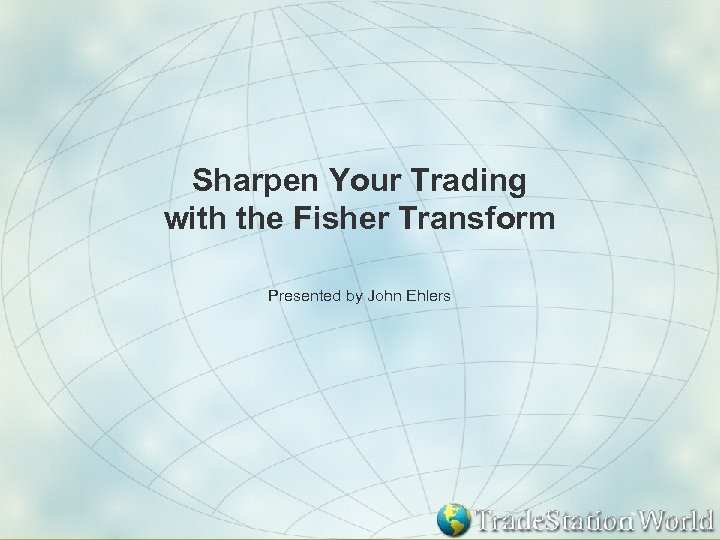Sharpen Your Trading with the Fisher Transform Presented by John Ehlers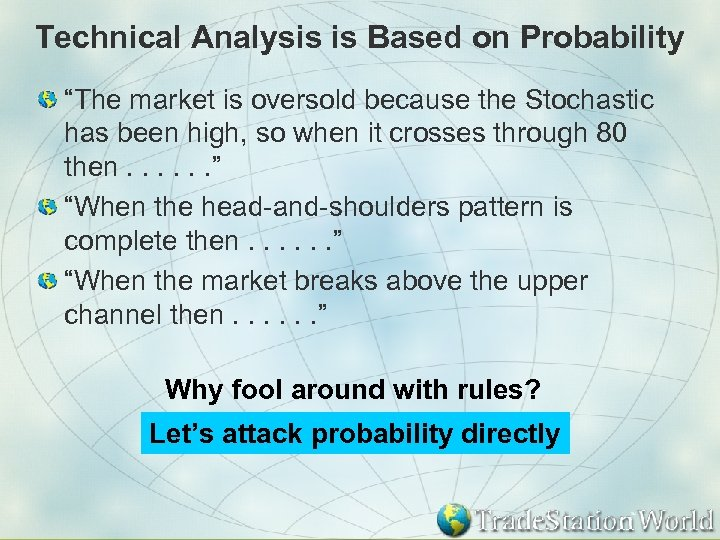Technical Analysis is Based on Probability “The market is oversold because the Stochastic has been high, so when it crosses through 80 then. . . ” “When the head-and-shoulders pattern is complete then. . . ” “When the market breaks above the upper channel then. . . ” Why fool around with rules? Let’s attack probability directly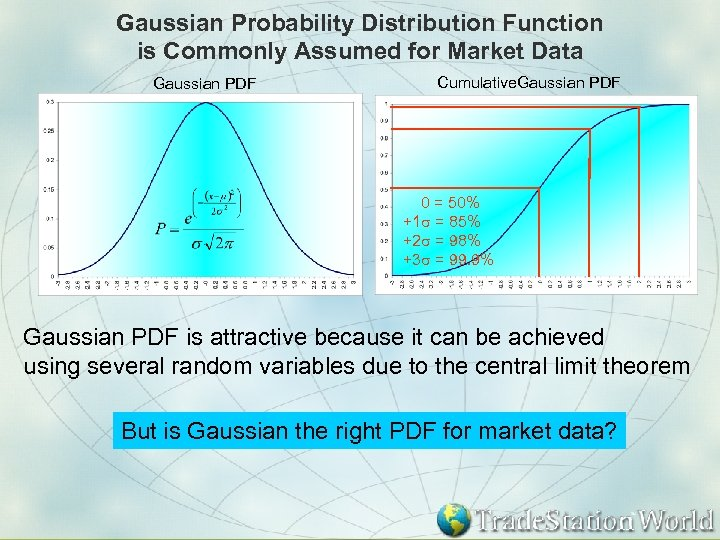Gaussian Probability Distribution Function is Commonly Assumed for Market Data Gaussian PDF Cumulative. Gaussian PDF 0 = 50% +1 s = 85% +2 s = 98% +3 s = 99. 9% Gaussian PDF is attractive because it can be achieved using several random variables due to the central limit theorem But is Gaussian the right PDF for market data?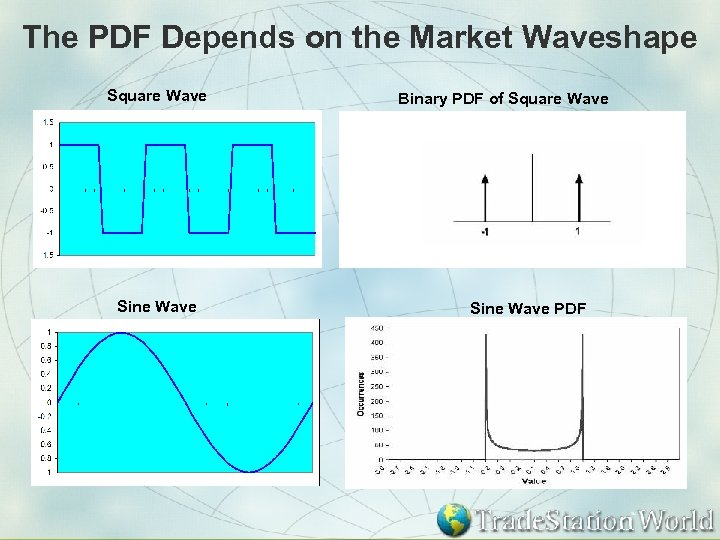The PDF Depends on the Market Waveshape Square Wave Sine Wave Binary PDF of Square Wave Sine Wave PDF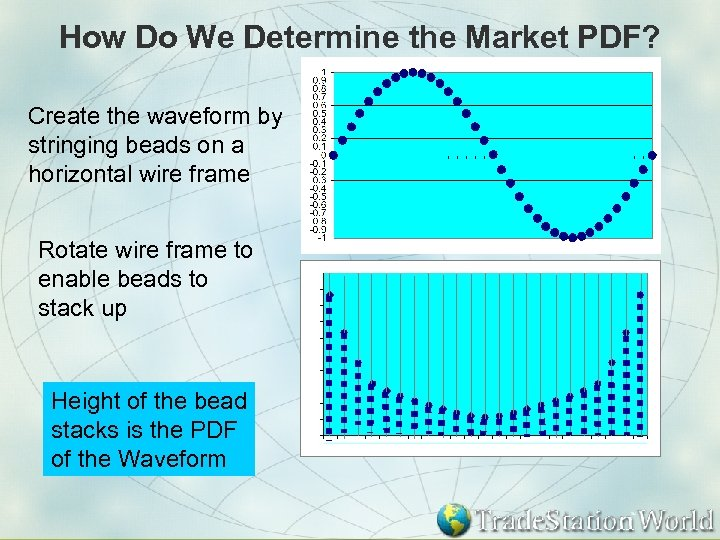How Do We Determine the Market PDF? Create the waveform by stringing beads on a horizontal wire frame Rotate wire frame to enable beads to stack up Height of the bead stacks is the PDF of the Waveform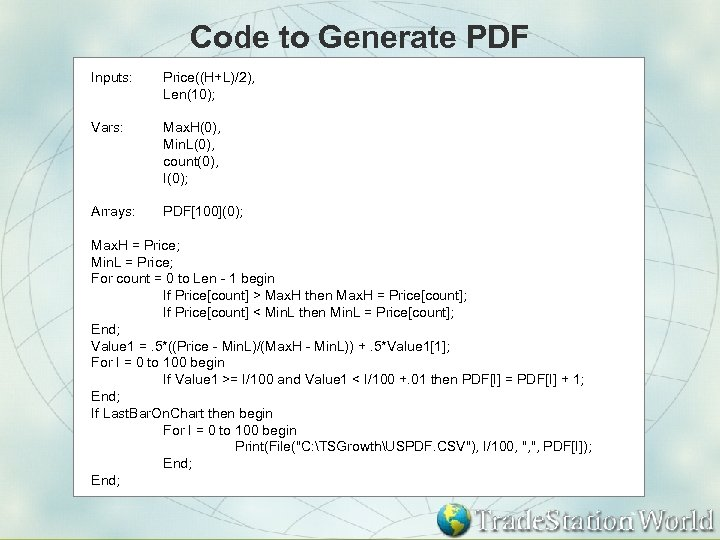Code to Generate PDF Inputs: Price((H+L)/2), Len(10); Vars: Max. H(0), Min. L(0), count(0), I(0); Arrays: PDF(0); Max. H = Price; Min. L = Price; For count = 0 to Len - 1 begin If Price[count] > Max. H then Max. H = Price[count]; If Price[count] < Min. L then Min. L = Price[count]; End; Value 1 =. 5*((Price - Min. L)/(Max. H - Min. L)) +. 5*Value 1; For I = 0 to 100 begin If Value 1 >= I/100 and Value 1 < I/100 +. 01 then PDF[I] = PDF[I] + 1; End; If Last. Bar. On. Chart then begin For I = 0 to 100 begin Print(File("C: TSGrowthUSPDF. CSV"), I/100, ", ", PDF[I]); End;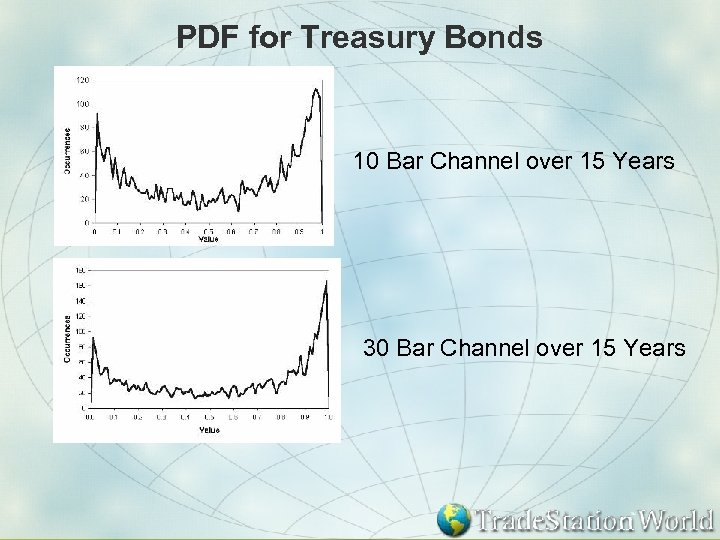PDF for Treasury Bonds 10 Bar Channel over 15 Years 30 Bar Channel over 15 Years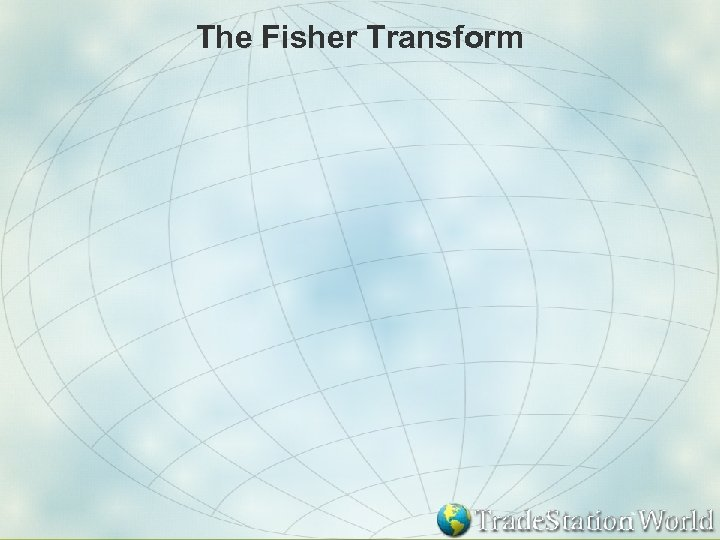The Fisher Transform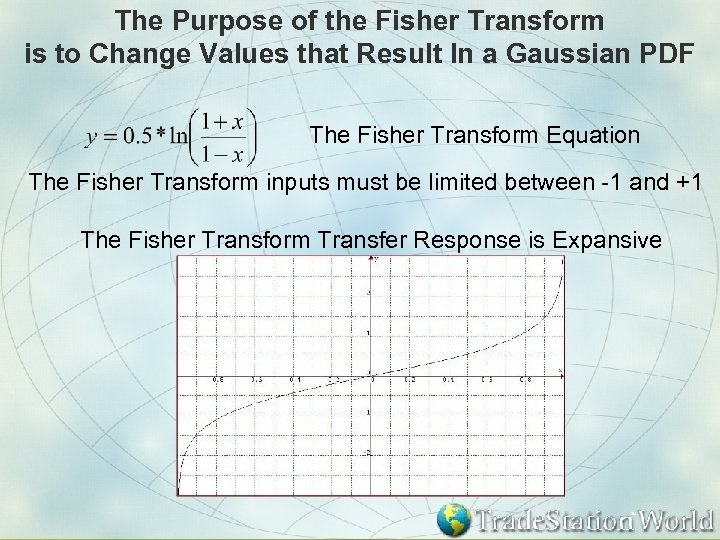The Purpose of the Fisher Transform is to Change Values that Result In a Gaussian PDF The Fisher Transform Equation The Fisher Transform inputs must be limited between -1 and +1 The Fisher Transform Transfer Response is Expansive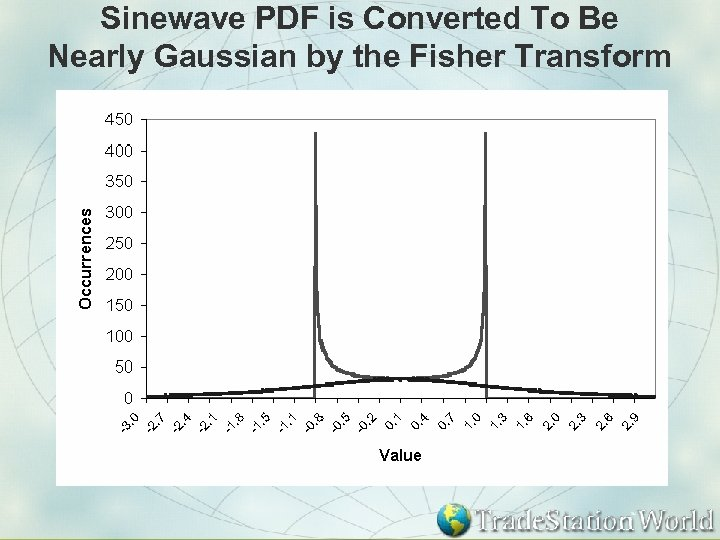Sinewave PDF is Converted To Be Nearly Gaussian by the Fisher Transform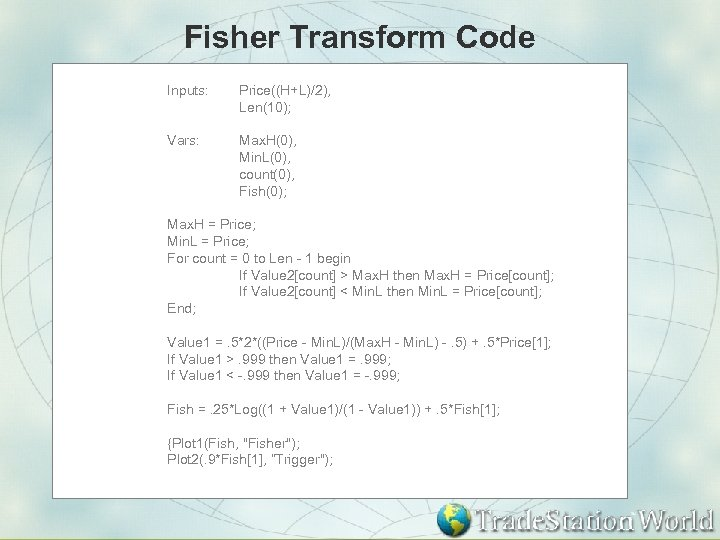Fisher Transform Code Inputs: Price((H+L)/2), Len(10); Vars: Max. H(0), Min. L(0), count(0), Fish(0); Max. H = Price; Min. L = Price; For count = 0 to Len - 1 begin If Value 2[count] > Max. H then Max. H = Price[count]; If Value 2[count] < Min. L then Min. L = Price[count]; End; Value 1 =. 5*2*((Price - Min. L)/(Max. H - Min. L) -. 5) +. 5*Price; If Value 1 >. 999 then Value 1 =. 999; If Value 1 < -. 999 then Value 1 = -. 999; Fish =. 25*Log((1 + Value 1)/(1 - Value 1)) +. 5*Fish; {Plot 1(Fish, "Fisher"); Plot 2(. 9*Fish, ”Trigger");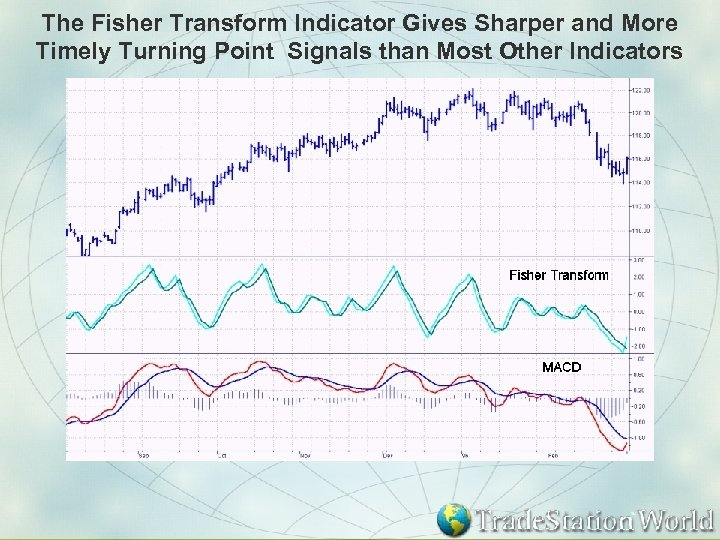The Fisher Transform Indicator Gives Sharper and More Timely Turning Point Signals than Most Other Indicators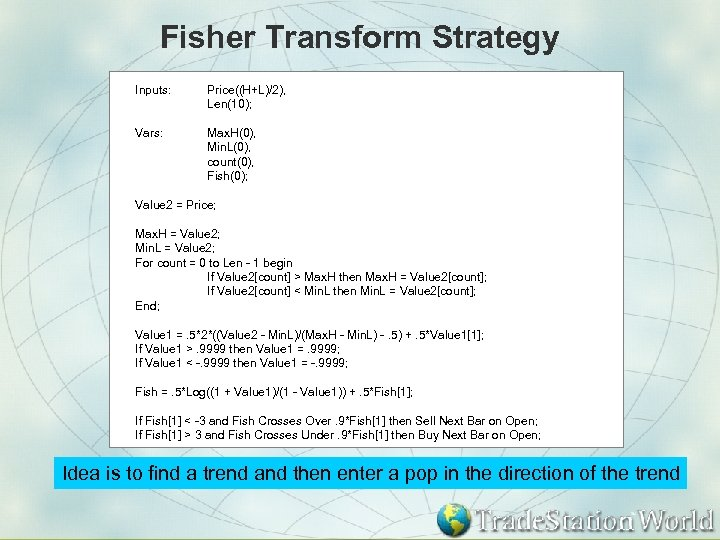Fisher Transform Strategy Inputs: Price((H+L)/2), Len(10); Vars: Max. H(0), Min. L(0), count(0), Fish(0); Value 2 = Price; Max. H = Value 2; Min. L = Value 2; For count = 0 to Len - 1 begin If Value 2[count] > Max. H then Max. H = Value 2[count]; If Value 2[count] < Min. L then Min. L = Value 2[count]; End; Value 1 =. 5*2*((Value 2 - Min. L)/(Max. H - Min. L) -. 5) +. 5*Value 1; If Value 1 >. 9999 then Value 1 =. 9999; If Value 1 < -. 9999 then Value 1 = -. 9999; Fish =. 5*Log((1 + Value 1)/(1 - Value 1)) +. 5*Fish; If Fish < -3 and Fish Crosses Over. 9*Fish then Sell Next Bar on Open; If Fish > 3 and Fish Crosses Under. 9*Fish then Buy Next Bar on Open; Idea is to find a trend and then enter a pop in the direction of the trend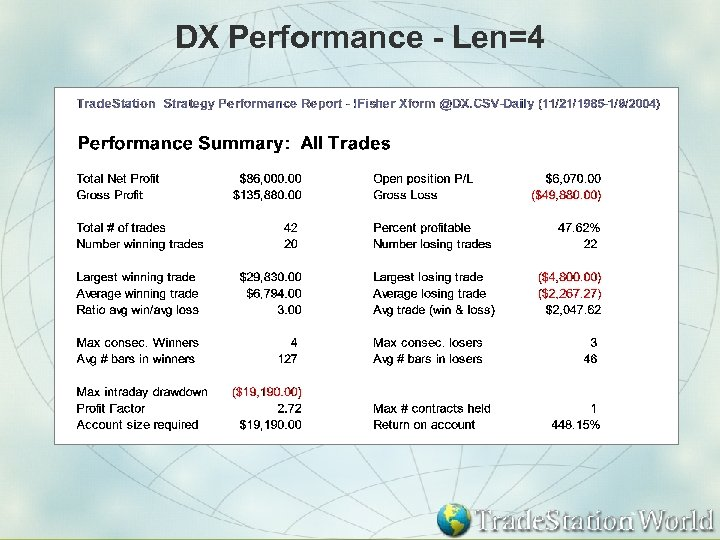DX Performance - Len=4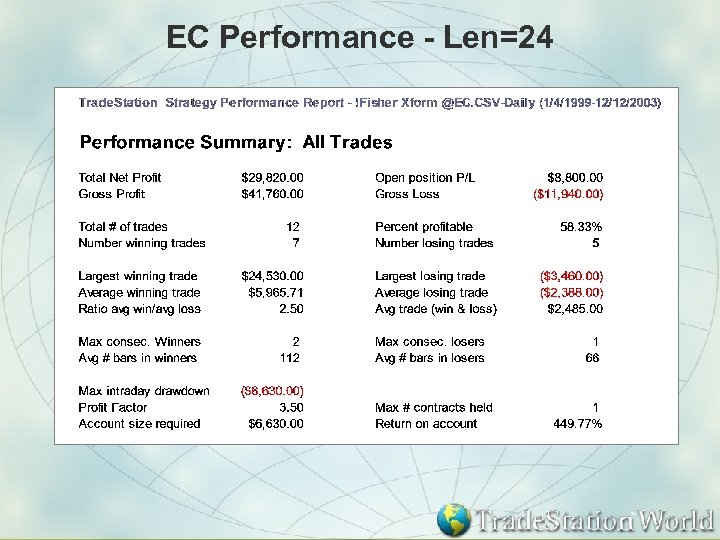EC Performance - Len=24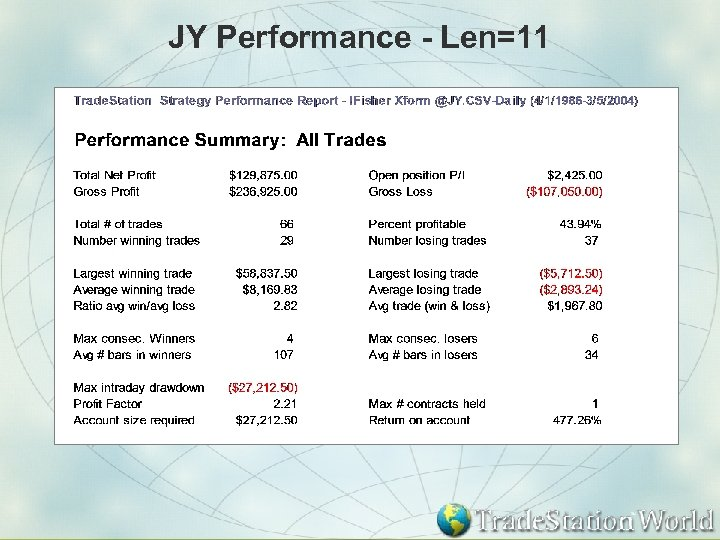JY Performance - Len=11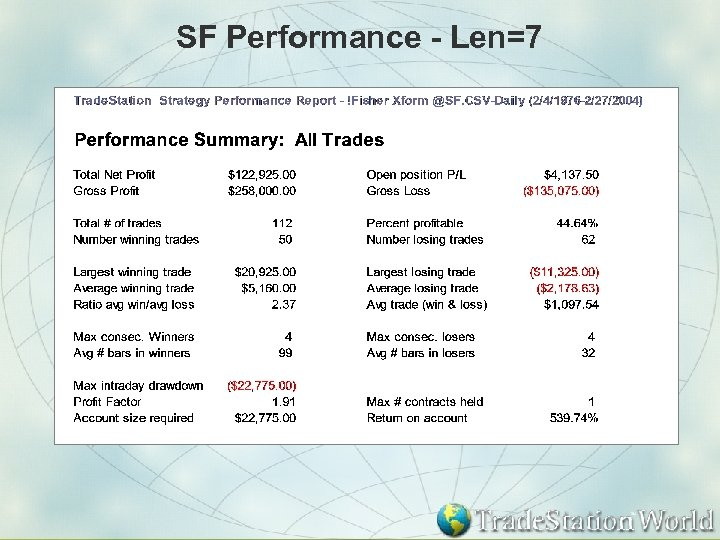SF Performance - Len=7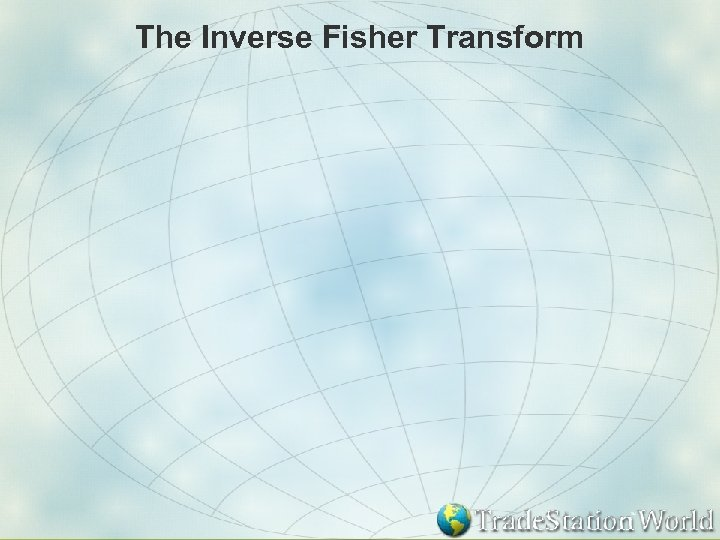The Inverse Fisher Transform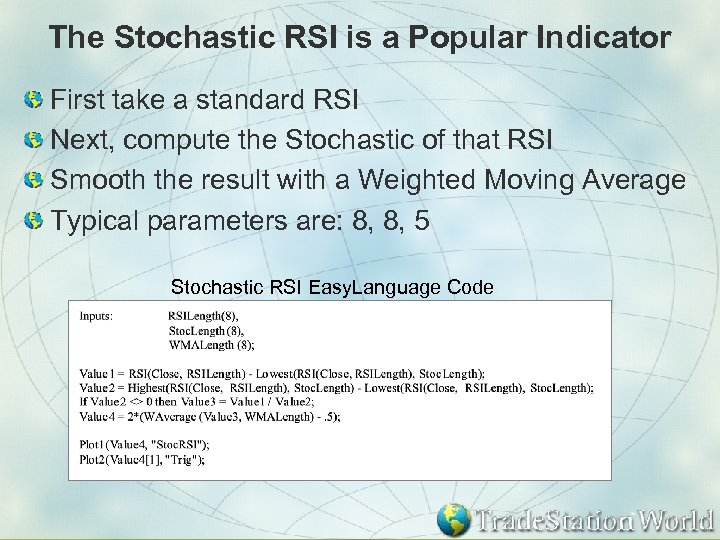The Stochastic RSI is a Popular Indicator First take a standard RSI Next, compute the Stochastic of that RSI Smooth the result with a Weighted Moving Average Typical parameters are: 8, 8, 5 Stochastic RSI Easy. Language Code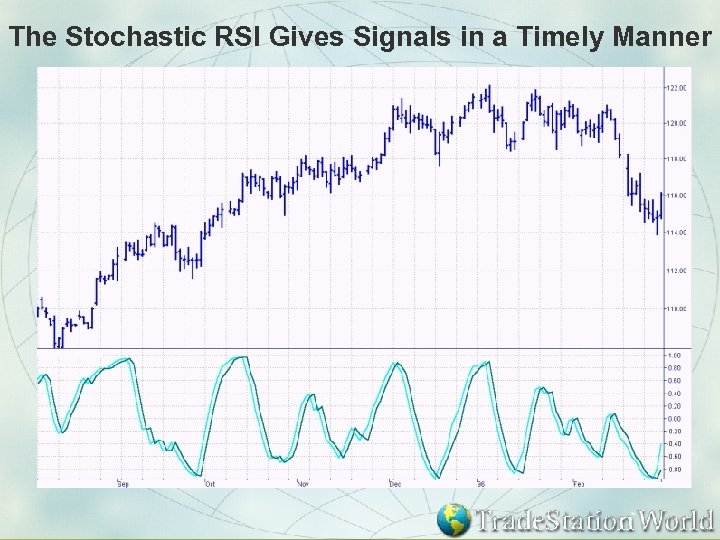The Stochastic RSI Gives Signals in a Timely Manner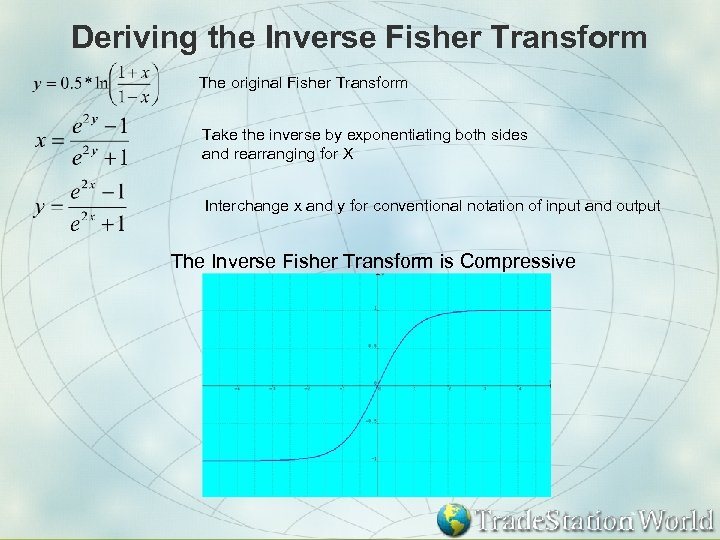Deriving the Inverse Fisher Transform The original Fisher Transform Take the inverse by exponentiating both sides and rearranging for X Interchange x and y for conventional notation of input and output The Inverse Fisher Transform is Compressive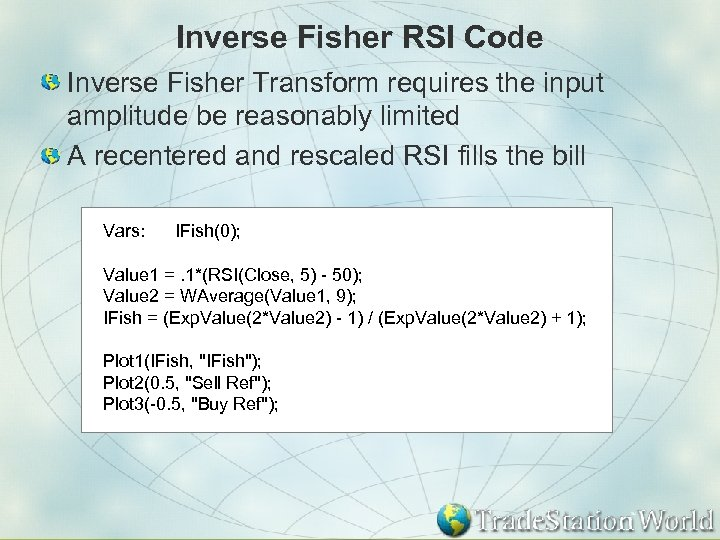Inverse Fisher RSI Code Inverse Fisher Transform requires the input amplitude be reasonably limited A recentered and rescaled RSI fills the bill Vars: IFish(0); Value 1 =. 1*(RSI(Close, 5) - 50); Value 2 = WAverage(Value 1, 9); IFish = (Exp. Value(2*Value 2) - 1) / (Exp. Value(2*Value 2) + 1); Plot 1(IFish, "IFish"); Plot 2(0. 5, "Sell Ref"); Plot 3(-0. 5, "Buy Ref");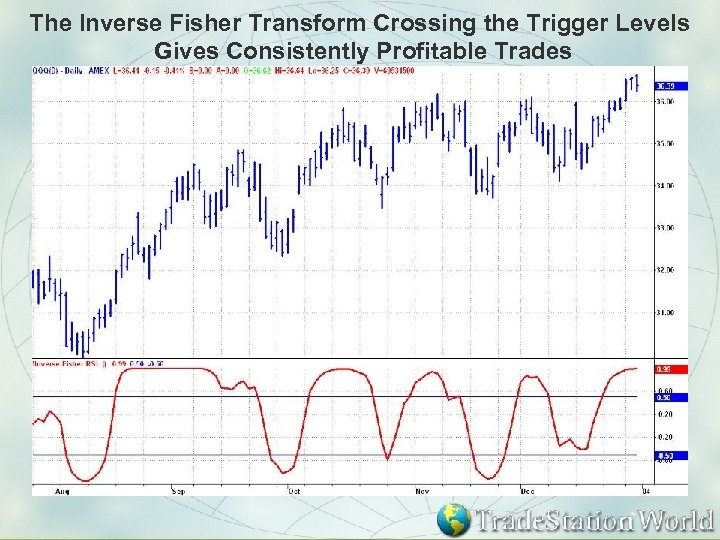The Inverse Fisher Transform Crossing the Trigger Levels Gives Consistently Profitable Trades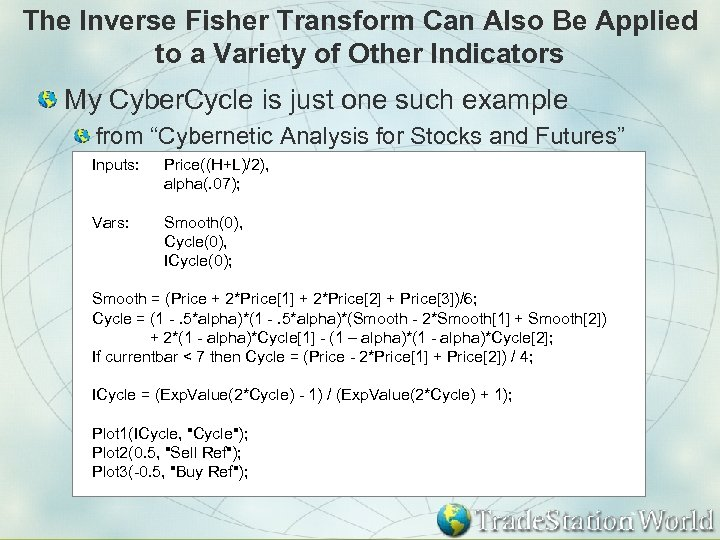The Inverse Fisher Transform Can Also Be Applied to a Variety of Other Indicators My Cyber. Cycle is just one such example from “Cybernetic Analysis for Stocks and Futures” Inputs: Price((H+L)/2), alpha(. 07); Vars: Smooth(0), Cycle(0), ICycle(0); Smooth = (Price + 2*Price + 2*Price + Price)/6; Cycle = (1 -. 5*alpha)*(Smooth - 2*Smooth + Smooth) + 2*(1 - alpha)*Cycle - (1 – alpha)*(1 - alpha)*Cycle; If currentbar < 7 then Cycle = (Price - 2*Price + Price) / 4; ICycle = (Exp. Value(2*Cycle) - 1) / (Exp. Value(2*Cycle) + 1); Plot 1(ICycle, "Cycle"); Plot 2(0. 5, "Sell Ref"); Plot 3(-0. 5, "Buy Ref");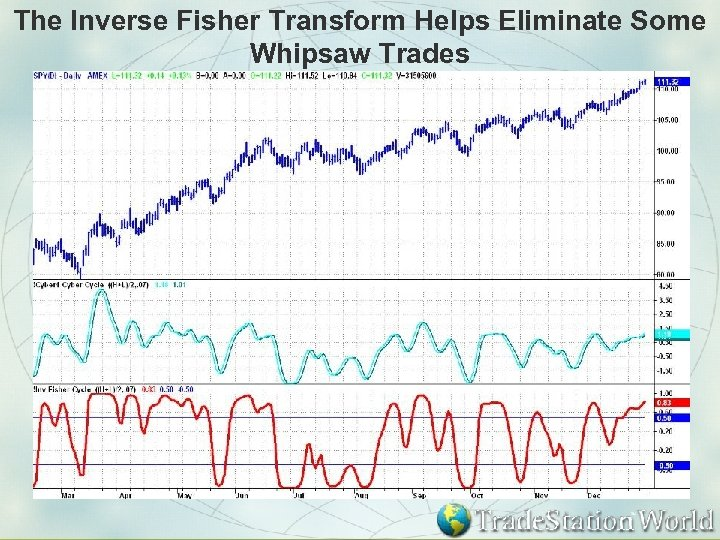The Inverse Fisher Transform Helps Eliminate Some Whipsaw Trades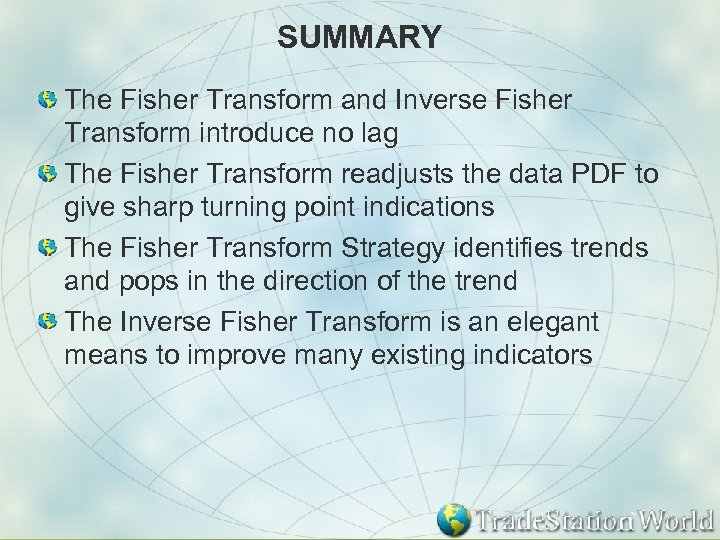SUMMARY The Fisher Transform and Inverse Fisher Transform introduce no lag The Fisher Transform readjusts the data PDF to give sharp turning point indications The Fisher Transform Strategy identifies trends and pops in the direction of the trend The Inverse Fisher Transform is an elegant means to improve many existing indicators